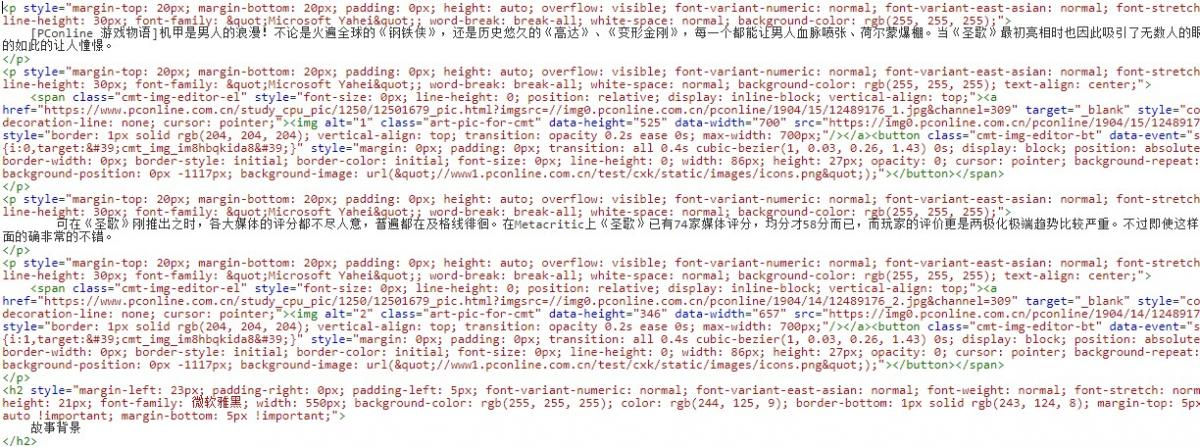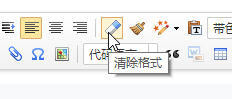# 批量清除所有文章的style样式（冗余代码）

## 前言## 批量清除代码

```<?php
require 'zb_system/function/c_system_base.php';

\$p = new Pagebar('{?page=%page%}', false);
\$p->PageCount = 30;
\$p->PageNow = (int) GetVars('page', 'GET') == 0 ? 1 : (int) GetVars('page', 'GET');
\$p->PageBarCount = \$zbp->pagebarcount;

\$articles = \$zbp->GetArticleList('*',array(array('search','log_Content','style=')),array('log_PostTime' => 'DESC'),array((\$p->PageNow - 1) * \$p->PageCount, \$p->PageCount),array('pagebar' => \$p));

foreach (\$articles as \$article){
\$article->Content = preg_replace('/[\s]{1}style="[\s\S].*?"/','',\$article->Content);
\$article->Save();
}

if (\$articles)
exit('已处理文章：'.(\$p->PageCount * \$p->PageNow).'<script>window.location.href="?page='.(\$p->PageNow + 1).'"</script>');
else
exit('操作完成');```